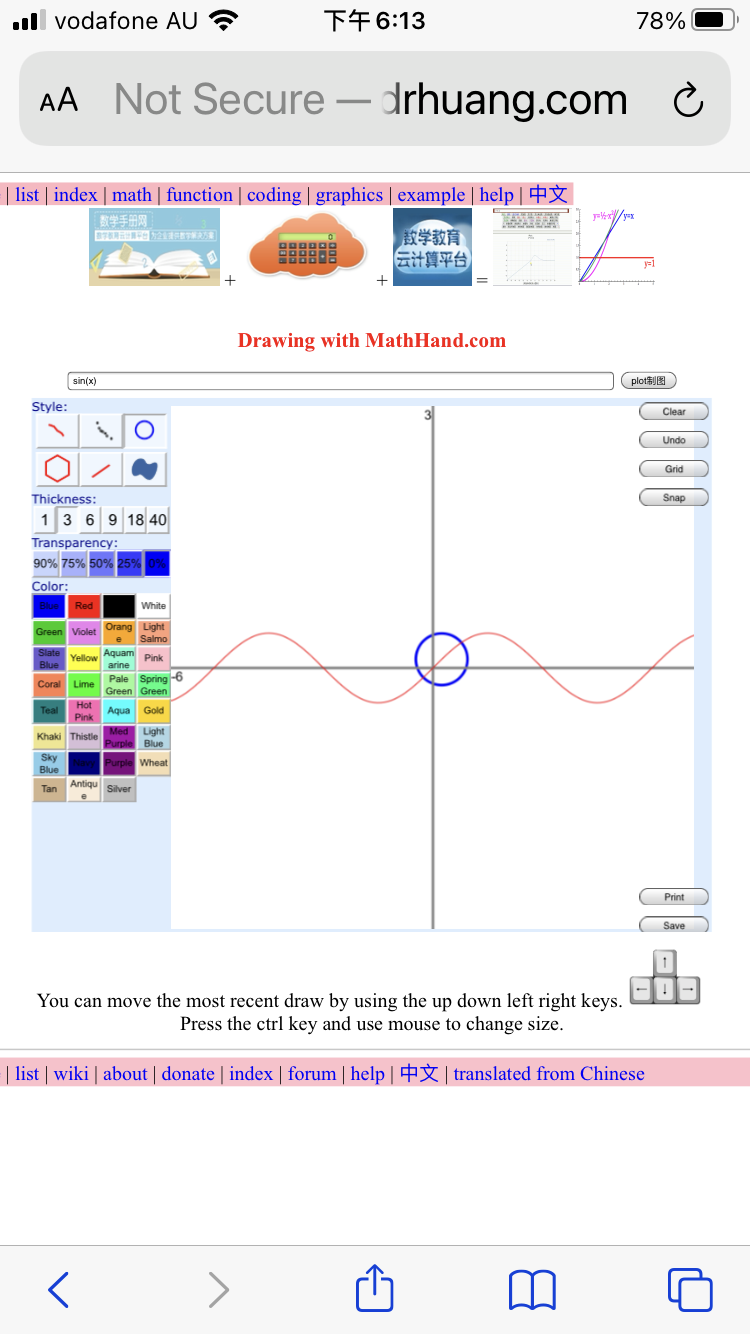﻿ ﻿ math handbook calculator - Fractional Calculus Computer Algebra System software+++=# Example of Graphics 制图

## on platform

### platform of Webpage editor

1. Webpage with Webpage editor

### platform of mathHand

2. mathHand coding.

### platform of mathHandbook

3. mathHandbook all-in-one calculator.

### platform of parametric plot

4. 200 surfaces of parametric function of real variable.
5. parametric3D

### platform of plot3D

6. plot3D(x) surface of function of real variable.

### platform of 3D surface plot

7. surface3D(x) surface of function of real variable.

### platform of 3D function plot

8. function3D(x) surface of function of real variable.

### platform of math3D

9. math3D surface of function of real variable.

### platform of complex plot

10. complexplot(z) surface of complex function of complex variable z.
11. complex animation(z) of complex function of complex variable z.
12. complex3D(x) surface of function of complex variable.
13. WebXR surface of complex function of complex variable.

### platform of draw

14. online drawing on platform draw with mouse, see Next =>

## Complex

1. complex - complex function - complex math
2. math handbook chapter 10 complex function
3. complex animate(z) for phase animation, the independent variable must be z.
4. complex plot(z) for phase and/or modulus, the independent variable must be z.
5. complex2D(x) for complex 2 curves of real and imag parts, the independent variable must be x.
6. complex3D(x) for 3 dimensional graph, the independent variable must be x.
7. color WebXR surface of complex function on complex plane
8. Riemann surface - Complex Branches - complex coloring